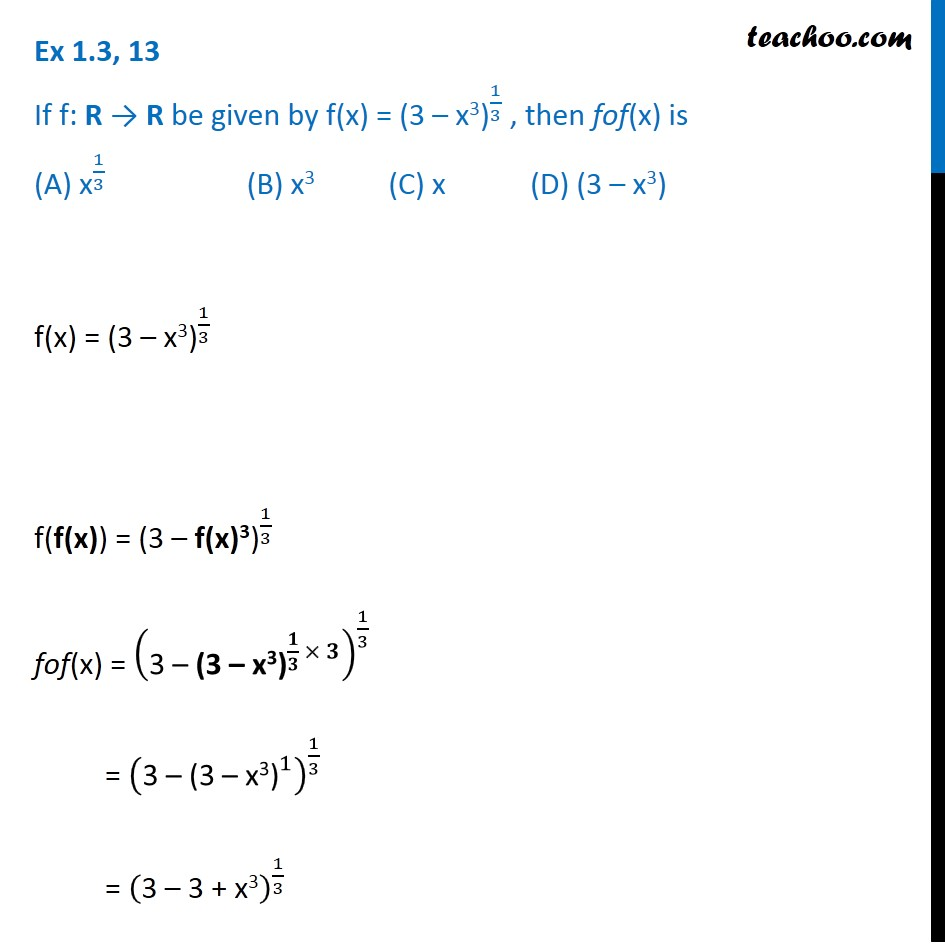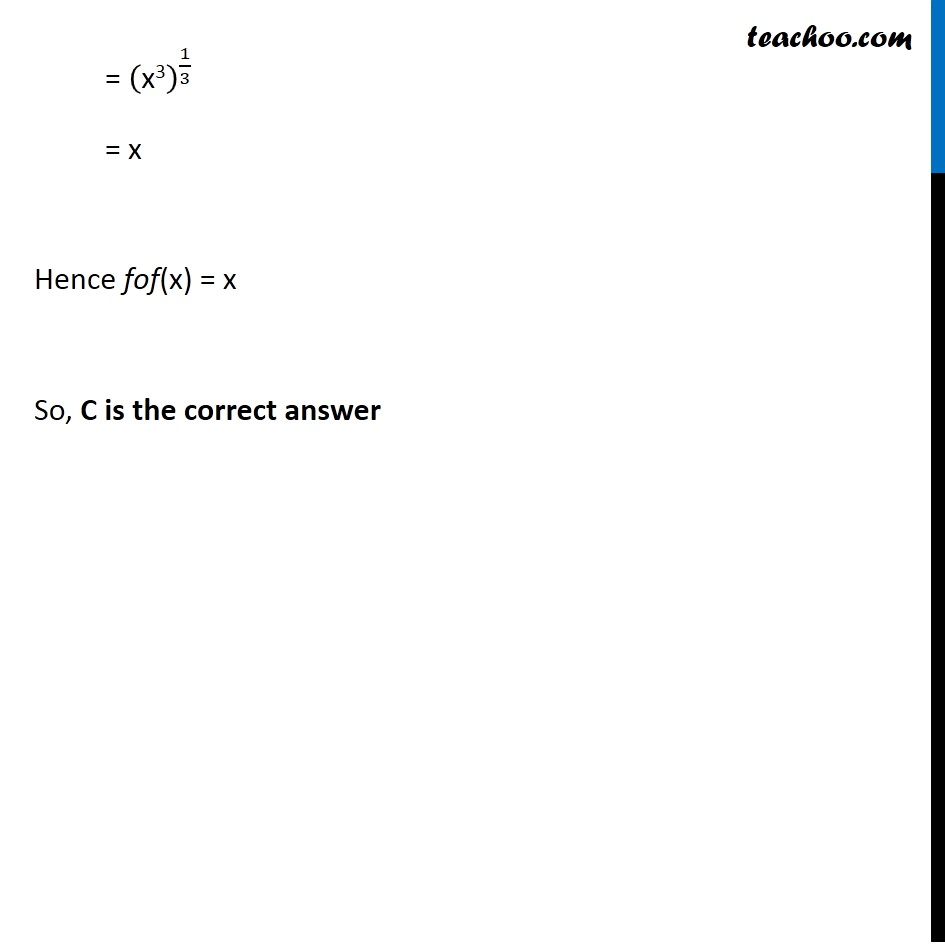Chapter 1 Class 12 Relation and Functions

Class 12
Important Questions for exams Class 12Introducing your new favourite teacher - Teachoo Black, at only ₹83 per month

### Transcript

Ex 1.3, 13 If f: R → R be given by f(x) = 〖"(3 – x3)" 〗^(1/3) , then fof(x) is (A) "x" ^(1/3) (B) x3 (C) x (D) (3 – x3) f(x) = 〖"(3 – x3)" 〗^(1/3) f(f(x)) = 〖"(3 – f(x)3)" 〗^(1/3) fof(x) = ("3 – " 〖"(3 – x3)" 〗^(𝟏/𝟑 × 𝟑) )^(1/3) = ("3 – " 〖"(3 – x3)" 〗^1 )^(1/3) = ("3 – 3 + x3" )^(1/3) = ("x3" )^(1/3) = x Hence fof(x) = x So, C is the correct answer